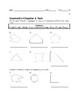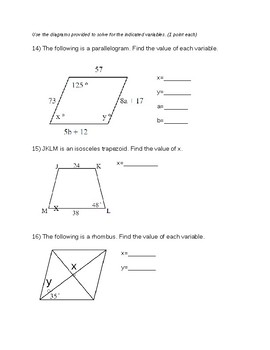# Remedial Geometry Test-Naming/Classifying Polygons, Angle Sums, QuadrilateralsSubject
Grade Levels
Resource Type
Product Rating
File Type

Word Document File

Be sure that you have an application to open this file type before downloading and/or purchasing.

376 KB
Share
Also included in:
1. Aligned with the Glencoe-McGraw Hill Geometry Textbook, these are tests over some of the tools of geometry including equations, plotting points, basic geometric structures, angles, lines, polygons, similar and congruent figures, the Pythagorean Theorem and trigonometry. These were intended for a rem
\$21.00
\$18.90
Save \$2.10
2. Aligned with the Glencoe-McGraw Hill Geometry Textbook, these are tests and quizzes over some of the tools of geometry including equations, plotting points, basic geometric structures, angles, lines, polygons, similar and congruent figures, the Pythagorean Theorem and trigonometry. These were intend
\$40.50
\$36.45
Save \$4.05
Product Description

Aligned with Chapter 6 of the Glencoe-McGraw Hill Geometry Textbook, this is a test over some of the tools of geometry including naming and classifying polygons, angle sums of polygons, and basic properties of quadrilaterals. It was intended for a remedial high school geometry class, so would be appropriate for middle-school aged students as well as a quick review for higher-level learners.

Total Pages
N/A
Answer Key
Not Included
Teaching Duration
N/A
Report this ResourceSign Up